# Introduction

The authors of this paper propose a new and simple technique to automatically design neural networks using policy gradients. The technique involves learning the optimal hyperparameters for an encoder-decoder network used for segmentation with the ACDC dataset. An optimal policy is found by iteratively perturbating a random policy and updating it with the perturbations that yielded the best reward (i.e. Dice index).

# Method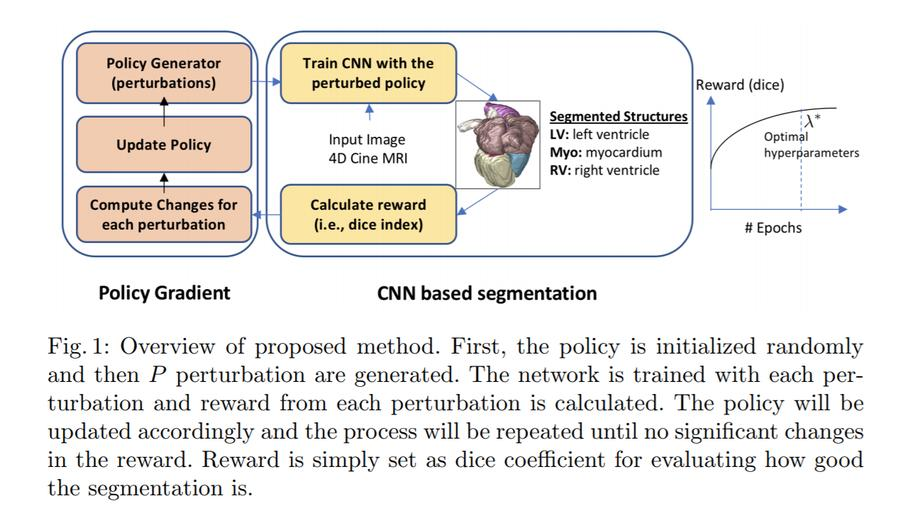In this method, each hyperparameter is considered a policy. The method starts with a base policy, initialized randomly.

Assume this random policy as $$\pi_0 = \{\theta_1, \theta_2, ..., \theta_N\}$$ indicating the hyperparamters of the network. N is the number of hyperparameters.

New policies are generated by applying random perturbations to the policy in each dimension (for each hyperparameter)

Let:

$P(\pi_0) = \{\pi_1, \pi_2,..., \pi_p\}$

define p random perturbations near $$\pi_0$$, where:

$\pi_i = \pi_0 + \Delta_i \text{ for } i \in \{1, 2,..., p\}$

For each perturbation $$\pi_i$$, $$\Delta_i$$ is defined as:

$\Delta_i = \{\delta^1, \delta^2,..., \delta^N\}$

where $$\delta^d$$ is a randomly chosen from $$\{-\epsilon^d, 0, +\epsilon^d\}$$ with $$d \in \{1, 2,..., N\}$$. $$\epsilon$$ is the derivative of a function $$y$$ with respects to $$x$$. These functions are the learnable hyperparameters for each layer:

• Number of filters: $$y_{NF} = 16x_{NF} + 16 \text{ with } x_{NF} = \{1, 2,..., 12\}$$
• Filter height: $$y_{FH} = 2x_{FH} + 1 \text{ with } x_{FH} = \{0, 1,..., 5\}$$
• Filter width: $$y_{FW} = 2x_{FW} + 1 \text{ with } x_{FW} = \{0, 1,..., 5\}$$
• Pooling function: $$y_{pooling} = x_{pooling} \text{ with } x_{pooling} = \{0, 1\}$$ where 0 is maxpooling and 1 is average pooling.

The segmentation network is trained with the p generated policies and a reward is obtained from each policy.

To estimate the partial derivate of the policy function for each dimension (hyperparameter), each perturbation is grouped to non-overlapping categores of negative perturbations, zero perturbations and positive perturbations. $$C^d_-$$, $$C^d_0$$ and $$C^d_+$$ such that $$\pi^d_i \in \{C^d_-, C^d_0, C^d_+\}$$. This categorization is based on the perturbation that was applied in that dimension ($$\{-\epsilon^d, 0, +\epsilon^d\}$$).

Then, the absolute reward for each category is calculated as a mean of all the rewards $$Ave^d = \{Ave^d_-, Ave^d_0, Ave^d_+\}$$ for each dimension d.

Finally, the initial policy is updated based on this average reward in the following way and the process is repeated:

$\pi^d_{0, new} = \begin{cases} \pi^d_0 - \epsilon^d & \text{if } Ave^d_- > Ave^d_0 \text{ and } Ave^d_- > Ave^d_+ \\ \pi^d_0 + 0 & \text{if } Ave^d_0 \geq Ave^d_- \text{ and } Ave^d_0 \geq Ave^d_+ \\ \pi^d_0 + \epsilon^d & \text{if } Ave^d_- > Ave^d_0 \text{ and } Ave^d_- > Ave^d_+ \\ \end{cases}$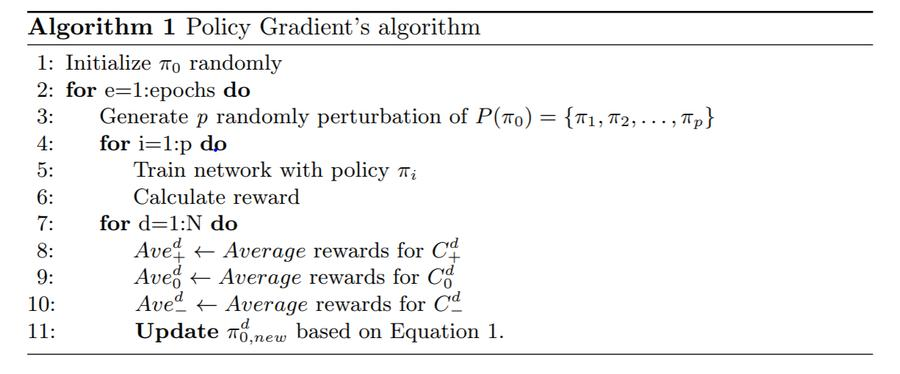# Architecture

This method was tested using a new architecture presented by the authors: densely connected encoder-decoder CNN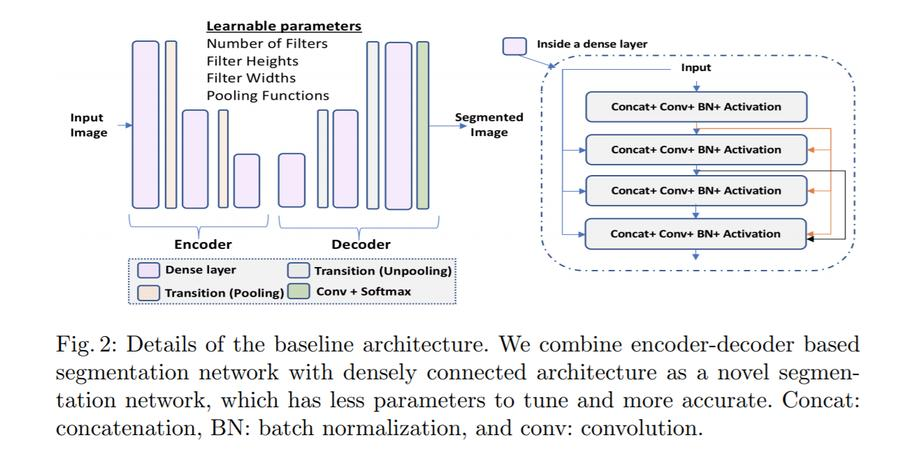Each layer of this architecture is defined by the following equation:

$F(X_l) = Conv(BN(Swish(X_l)))$

Because each layer is connected to all previous layers in this architecture, each layer is further defined as:

$F(X_l) = F( \parallel_{l' = 0}^{l'=l-1} F(X_{l'})) \text{ for } l \geq 1 \text{ and } l = \{1, 2,...L\}$

where $$\parallel$$ is the is the concatenation operation along the channel axis.

The encoder’s downsampling layers are either max or average pooling (learned by the policy gradient).

There are therefore 76 hyperparameters.

• 3 parameters for each of the 25 layers (the last layer has a fixed number of filters)
• 2 additional hyperparameters for the 2 pooling layers.

# Experiments

This method and architecture were tested on the ACDC dataset. The network was trained with an Adam optimizer with learning rate 0.0001 and a cross entropy loss. Each perturbated network was trained for 50 epochs in order to evaluate the reward (average over the last 5 epochs).

The authors used rotations and scaling to augment the data and a 3D fully connected Conditional Random Field (CRF) to refine their results.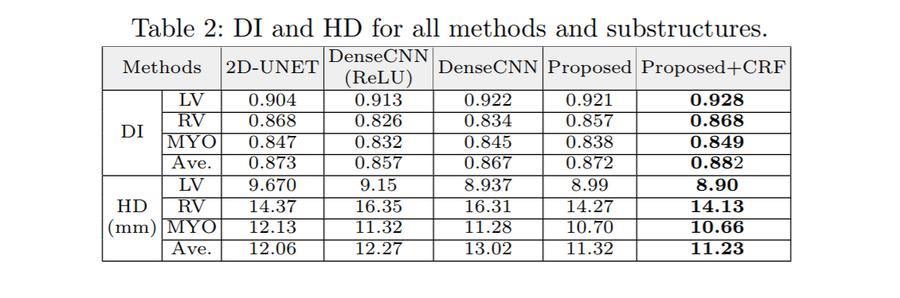The final learned hyperparameters are: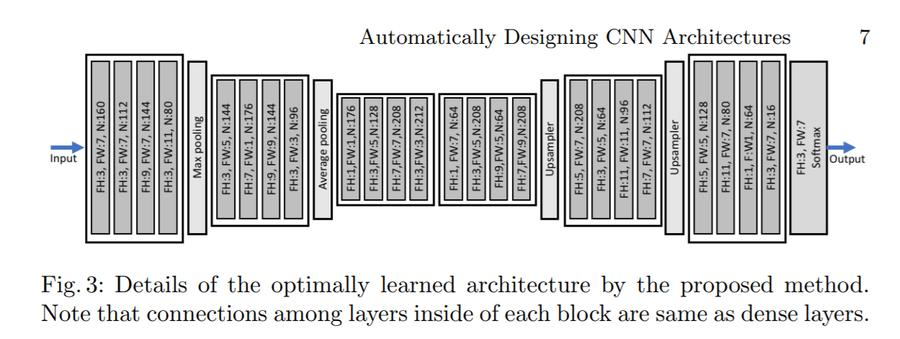The method was trained on 15 Titan X GPUs for 10 days.